Thank you for visiting nature.com. You are using a browser version with limited support for CSS. To obtain the best experience, we recommend you use a more up to date browser (or turn off compatibility mode in Internet Explorer). In the meantime, to ensure continued support, we are displaying the site without styles and JavaScript.

# A measure of individual role in collective dynamics

## Abstract

Identifying key players in collective dynamics remains a challenge in several research fields, from the efficient dissemination of ideas to drug target discovery in biomedical problems. The difficulty lies at several levels: how to single out the role of individual elements in such intermingled systems, or which is the best way to quantify their importance. Centrality measures describe a node's importance by its position in a network. The key issue obviated is that the contribution of a node to the collective behavior is not uniquely determined by the structure of the system but it is a result of the interplay between dynamics and network structure. We show that dynamical influence measures explicitly how strongly a node's dynamical state affects collective behavior. For critical spreading, dynamical influence targets nodes according to their spreading capabilities. For diffusive processes it quantifies how efficiently real systems may be controlled by manipulating a single node.

## Introduction

Complex networks are a groundbreaking concept that is helping to understand the behavior of many chemical, biological, social and technical systems1,2. Network representations are particularly suitable for systems where heterogeneity dominates and are crucial for dynamics3, where a few nodes are usually considered as the most important. Oftentimes, node importance is correlated with centrality measures, local4,5 or global6, which usually do not explicitly account for the dynamics as they are generally based on a purely topological perspective. However, dynamics is fundamental in assessing the impact of individual elements in global performance and in controllability problems7. Here, we show that dynamical influence is a centrality measure able to quantify how strongly a node's dynamical state affects the collective behavior of a system, taking explicitly into account the interplay between structure and dynamics in complex networks. We prove that it applies equally well to a variety of families of dynamical models, from spreading phenomena at the critical point to diffusive processes and and continuous-time dynamical system such as the Kuramoto model and the Roessler chaotic dynamics.

Classical centrality measures in complex networks –like the degree or number of neighbors a node interacts with4,5, betweenness centrality8 counting the number of shortest paths through a certain node, eigenvector centrality9 based on the idea that relations with more influential neighbors confer greater importance, or the k-shell decomposition10 that correlates with the outcome of supercritical spreading originating in specific nodes11,12,13– rely only on topology, even if an underlying process can be indirectly associated in some cases. In contrast, the impact of individual elements in the global performance of the system inevitably depends on the specificities of the dynamics. Targeting individuals for vaccination strategies in epidemic processes is not the same as selecting electrical stimulation sites in the brain in order to suppress epileptic seizures. In this respect, a Laplacian-based centrality measure14,15,16, closely related to PageRank17, has been proposed recently to assess the importance of complex network nodes in specific dynamical models.

In this work, we provide a general and rigorous framework where dynamical influence is defined as a centrality measure both on directed and on undirected complex networks and applies to a variety of families of dynamical models, including epidemic spreading models like the susceptible-infected-removed (SIR), the susceptible-infected-susceptible (SIS) and the contact process, the Ising model and diffusive processes like the voter model or phase coupled oscillators. In all cases, dynamical influence is calculated as the leading left eigenvector of a characteristic matrix that encodes the interplay between topology and dynamics.

## Results

### Defining dynamical influence

We focus on systems of N time-dependent real variables x = (x1, …, xN) with coupled linear dynamics specified by a N × N real matrix M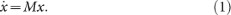A first classification of the dynamics is obtained by considering the largest eigenvalue µmax of M. For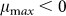, x(t) converges to a null vector that represents a stable fixed point solution; for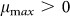, indefinite growth from almost all initial conditions is observed. Suppose that M is such that a non-degenerateexists. Then, the scalar product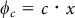is a conserved quantity, where c is the left eigenvector of M for µmax,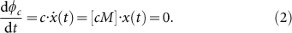The existence of the conserved quantity allows to calculate the final state in terms of the initial condition x(0) as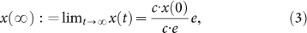where e is a right eigenvector of M for µmax. This equation implies that the projection of x(0) on c is all the system remembers at large times about the initial condition x(0). The coefficient ci quantifies the extent to which the initial condition at node i affects the final state. Therefore, we call ci the dynamical influence (DI) of element i on the dynamics under equation (1).

One advantage of DI is that it is easily calculated without expensive numerical simulations. In fact, a simple way to calculate c furthers the understanding why this object quantifies the role of nodes in spreading dynamics. The power method (also called power iteration)18 approximates c by applying higher and higher powers of M to a uniform vector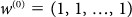. For general exponent, the i-th entry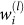ofis the number of all possible walks of length l departing from node i or, in other words, the number of ways an item can spread for l steps when originating at node i. At the first iteration this yieldswhere di is the sum over the i-th row of M. When M is the adjacency matrix of a network, then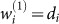is the (out-)degree, the number of (outgoing) connections of node i. For exponent 2, the i-th entry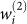is the sum of the (out-)degrees of all neighbors of i. This is the same as the number of possibilities (walks) to depart from node i following two links. Now in the limit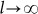, the direction of the eigenvector c is approached by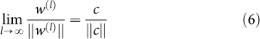when the largest eigenvalue of M is non-degenerate and larger in magnitude than the other eigenvalues. Hence, the dynamic influence ci of element i is its ability to serve as the origin of many arbitrarily long walks on the network.

Let us first apply these insights to critical phenomena like spreading processes19. In the SIR model20,21,22, each node is either susceptible, infected or removed. An infected node i transfers the epidemic along each of its outgoing arcs independently with probability β; node i itself relaxes to the removed state at unit rate. We study small perturbations to the stationary state with all nodes susceptible and approximate the dynamics by the linearization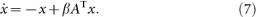Here xj(t) is the probability of node j to be infected at time t. The first term is the relaxation from the infected to the removed state at unit rate. The second term quantifies the transmission of the epidemic where the network enters by the transpose of its adjacency matrix A.

Equation (7) can be rewritten as equation (1) with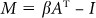and I being the identity matrix. Matrix M has largest eigenvalue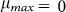when the spreading probability β is the inverse of the largest eigenvalue of A, that is. We take again c as a left eigenvector of M ator, equivalently, a right eigenvector for maximum eigenvalue αmax of A. Then the expected outbreak size from an initial infection described by the probability vector x(0) is proportional to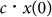.

Now we ask how well c may forecast the actual SIR spreading dynamics, measured as the spreading efficiency (details in Methods) of node i that we define as the expected fraction of nodes reached by an epidemic outbreak initiated with node i infected (seed node), all others susceptible. Figure 1 shows that ci is a good predictor of SIR spreading efficiency at critical parameter value β = βc in a small social network. Dynamical influence ci outperforms the predictions made by degree, shell index and betweenness centrality. Predictive power is quantified by the rank order correlation (see Methods).

Figure 2 shows the predictive power of dynamical influence for spreading efficiency as a function of the infection probability in larger real-world networks and the Barabasi-Albert model. The results are as anticipated by the theory. Dynamical influence is a good predictor of spreading efficiency in the critical regime where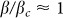. Predictions by dynamical influence outperform those by other quantities that are supposed to provide information about expected outbreak size in a broad interval of infection probabilities. This still holds for values of β that lead to average outbreak sizes of up to 10% of all nodes in the network, as indicated by the vertical dashed lines in Figure 2.

The approximation w(l) for finite length l in Eq. (4) is useful as a predictor of spreading efficiency as well. Even when the interaction network is not completely available, local information counting the number of walks of length l = 2 or l = 3 emanating from a node is enough to estimate dynamical influence. Figure S1 in the Supplementary Information shows that the count of these short walks yields a prediction of spreading efficiency in the critical regime that is as good as dynamical influence itself. The predictive power of these walk counts, too, reaches a maximum in the critical regime.

For infection probabilities β far above or below the critical value βc, however, the degree di of a node i is a better predictor of spreading efficiency. In the subcritical regime, spreading is sparse and typically confined to the neighborhood of the seed node i, while in the supercritical regime, the epidemics rarely fails to spread to the whole system. In the critical regime in-between these extremes, infectious seeds are perturbations that trigger relaxation dynamics at all scales. This is reflected in a dynamics dominated by a marginal linear mode and a variety of possible final states. Dynamical predictions at criticality require then a global view of the network structure (and the final state is determined by the conservation law associated with the leading eigenvector c-removed). The scale-free distribution of epidemic outbreaks in real populations23,24 is a sign of criticality and suggests that this regime is most relevant in practice.

In order to check the robustness of the results we consider two modifications of the epidemic spreading dynamics. First, we study the SIR model with a stochastic rather than deterministic transition from the infected to the removed state. Specifically, the transition occurs with a probability µ independently for each infected node at each time step. Thus the time spent in the infected state (recovery time) has a geometric distribution with mean µ–1. This modification does not qualtitatively change the results of Figure 2 up to rescaling of β with µ. In fact, the curves of predictive power for different values of µ collapse when plotted as a function of the average outbreak size, see Figure S2. Second, prediction by dynamical influence may also be applied to the SIS model (see Materials and methods) yielding very similar results (Supplementary Information, Figure S3). The contact process25 can also be considered, with A replaced by the stochastic adjacency matrix, the adjacency matrix after normalization such that each row sums up to 1.

To facilitate intuitive understanding of the predictive role of centrality measures in spreading dynamics, let us consider small networks. In each of the three cases in Fig. 3, a different subset of the measures yields the correct ranking by spreading efficiency at the critical point. The most efficient spreader is not necessarily the node with the largest degree. Being adjacent to several nodes with large degree may lead to large spreading efficiency despite a smaller degree, cf. panel (a). This second order effect is reflected by dynamical influence. When all degrees are equal as in panel (c), also dynamical influence and shell index are homogeneous. In this case, betweenness centrality captures the subtle effect of nodes having different positions in the network. We speculate that centrality measures based on unconstrained walks and shortest paths can do best in predicting spreading efficiency at the critical point. Then, a suitable combination of dynamical influence with betweenness depending on network topology might be close to the optimal predictor.

Nodes with large shell index are contained in highly connected neighborhoods that facilitate spreading. In many cases, the shell index may serve as a satisfactory predictor of spreading efficiency13. Here, however, we find situations where its use for prediction is limited due to the degeneracy of the values shell index assumes. It has a constant value m across nodes of each network that can be built up by iterative attachment of a node with exactly m edges. This includes all trees (m = 1) and networks from growth models such as the one by Barabasi and Albert4. A significant lack of resolution is also observed in real-world networks. Shell index assumes only few (≈ 10) discrete values, cf. Figure S4 in Supplementary Information. On the Internet graph, the same maximum shell index value is observed for the strongest spreader as well as nodes with spreading efficiency a factor of five below. Thus, even though the overall correlation between spreading efficiency and shell index is positive, lack of resolution limits the predictive power. Such limitations have been identified also in an empirical study of epidemic spreading in a social group26, in a detailed comparison between SIR and SIS models27 and in dynamics of rumour propagation28.

We remark that the most efficient spreaders are not necessarily the same as those targeted by efficient vaccination strategies in order to contain epidemics. At the network level, the aim of vaccination is to increase the epidemic threshold β in order to render the spreading dynamics subcritical. The set of nodes by whose removal this shift of threshold is achieved29 is different in general from the set of nodes with the largest dynamical influence. The Supplementary Information provides further results (Figure S5) and a brief discussion of vaccination.

### Ising model

The Ising model is a paradigmatic binary state model of critical phenomena. The Ising model30,31 on a network32 describes the dynamics of N coupled spins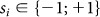placed on the nodes. The zero temperature (T = 0) version of the Ising model implements a majority rule for state updating. This is the same dynamics considered in threshold models of collective behavior for a 50% value of the threshold33,34 and its dynamics is also related to Schelling's model of urban segregation35,36; the finite temperature version has been considered in the context of strategic interactions37. Finite temperature effects (noise), as considered here, are essential to escape from frozen configurations and to establish the robustness of transitions found in Ising-like models38. Also in the theory of neural computation, Ising-like systems play an essential role39.

In the context of the Ising model, we define spreading efficiency of node i as the correlation between two measurements: the state of node i at time t and the magnetization (see Methods) of the whole system at a later time, formally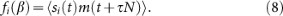The parameter τ measures the time lag between the two measurements. Figure 4 shows to which extent the ranking of nodes by Ising spreading efficiency is correlated with the ranking by various centrality measures. At the transition between order and disorder, Ising spreading efficiency has larger correlation with dynamical influence than with the other centrality measures.

### Diffusive processes: the voter model

Coming back to the general framework equation (1), there is a class of dynamical processes in networks in which the property of M having a zero maximum eigenvalue appears naturally without the need of adjusting any parameter. This is the case of diffusive processes defined by equation (1) with M = −L and the Laplacian matrix entries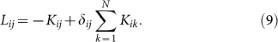The zero eigenvalue of L is non-degenerate under mild assumptions40. For these processes our general analysis of equation (1) becomes exact. A prominent example of diffusive dynamics is the voter model41 in which node i is in a spin state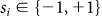. For this model, xi stands for the ensemble average of spin i,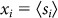and Kij gives the rate at which node i copies the state of node j. Different definitions of the voter model dynamics provide clear examples of how the concept of dynamical influence takes into account the interplay between topology and dynamics: For link update dynamics in an undirected network, an ordered pair of nodes (i, j) is chosen in each step and node i copies the state of node j. The rate matrix K becomes proportional to the transpose of the adjacency matrix A. As a consequence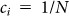, the average magnetization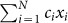is conserved and all nodes have the same dynamical influence independently of the topological features of the network. In the more standard node update voter dynamics, at each step one node i (having degree di) is selected at random and copies the state of one of its neighbors j, also selected at random. In this case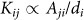, so that Kij is no longer a symmetric matrix, the conserved quantity is a weighted magnetization42 and the dynamical influence of node i is proportional to its degree di.

For diffusive processes, the system is driven towards a homogeneous final state with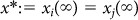for all i and j. Although x* takes continuous values, each realization of the voter dynamics in a finite system eventually reaches a homogeneous absorbing state with either all nodes in the state +1 or all in the state −1. The influence ci of a node weights the initial state of node i in the exit probability P+, that is, the probability to reach the absorbing configuration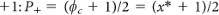. When all nodes are equivalent (e.g., link update) x* is just the average of the initial values of the nodes, but otherwise (e.g. node update) x* is given by a weighted average of the initial condition. The value ci has an alternative interpretation as a stationary density of a random walk15.

### Efficient driving of complex systems

The meaning of dynamical influence also manifests itself in the practical task of driving a system efficiently. In the context of the voter model, this task might be phrased in terms of the zealot problem43. One considers a special directed network in which a given node (the zealot) does not copy the state of any of its neighbors. The question is the efficiency of the zealot in driving all other nodes to the zealot state. To show the broad applicability of the dynamical influence concept, we address this question of driving efficiency considering the problem of phase-coupled oscillators described by the Kuramoto model44. Assuming all oscillators have the same intrinsic frequency ω (without losing generality, we choose ω = 0), the phase variable xi of oscillator i advances as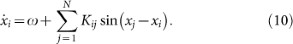with a matrix K of non-negative coupling strengths. Around the synchronized state, phase differences are small. By approximating each sin-term with its argument, a linear homogeneous system as in equation (1) is recovered.

We study a scenario with initially all oscillators i in phase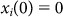. An additional node a with constant phase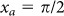is added to the system and linked through an additional edge to a chosen node i. We measure the time Ti the system takes to reach the new homogeneous state with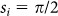for all nodes i. The dynamical evolution of these systems is illustrated by studying the motif in the inset of Fig. 5a. The global phase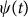converges faster to the external forcing when the driving is applied to the nodes with higher influence and the convergence of the different nodes depends on their relative network position in relation to the driver. In Fig. 5(b), we show the results on a directed network of phase oscillators connected as the network of regions in the macaque cortex45. Dynamical influence has extremely high predictive power. The rank order correlation of driving efficiency with dynamical influence is 0.97, while 0.66 with degree ratio, −0.14 with shell index and −0.09 with betweenness. Similar results are obtained on randomly grown directed networks and for coupled chaotic oscillators, see Figures S6 and S7 in Supplementary Information. These findings clearly show that dynamical influence is an excellent proxy to identify better targets for controlling global behavior, even in strongly non-linear dynamical systems.

## Discussion

Dynamical influence is a centrality measure applicable to a wide range of dynamical processes on complex networks that takes into account the interplay between topology and dynamics. While the motivation and rigorous analysis of dynamical influence employ the context of linear systems, its practical use for understanding and controlling networked dynamics extends to several inherently non-linear systems.

We have demonstrated that dynamical influence is applicable to stochastic equilibrium (Ising model) and nonequilibrium systems (epidemic and voter models) as well as deterministic state-continuous systems such as the Kuramoto model and the chaotic Roessler attractor. For critical epidemic spreading and the Ising model, dynamical influence is a good predictor of spreading capabilities. In the context of chaotic Boolean dynamics46, a similar spectral centrality is highly correlated with a node's impact on the attractor reached47. For diffusion, dynamical influence quantifies the impact of the dynamical states of single nodes on the asymptotic homogeneous state. Beyond that, it proves to be a high-quality proxy for driving efficiency, uncovering which are the best target nodes in real networks to be forced in order to drive the system towards specific states. In a broader context, the identification of these targets has fundamental implications and practical applications on strategies with an interest in controlling collective behavior, from social influence to biomedical responses.

## Methods

### Epidemic models

We simulate the SIR model of epidemic spreading in the time-discrete version. Transitions between the three states (S,I,R) are as follows. If node i is in the S (susceptible) state and has ν infected (I) neighbors at time t, then node i remains susceptible with probability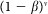, otherwise i is infected at time t + 1. If node i is in the infected state at time i then i is in the R (removed) state at time t + 1. In the SIS model, at difference with SIR, a node infected at time t is susceptible again at time t + 1. The probability of being removed in the SIR model does not enter in the linearized equation (7) because it appears only in a second order term in the equation for x. Therefore equation (7) gives the same linear description for the SIR and SIS models.

The system is in an absorbing configuration if none of the nodes is infected. For both models, outbreak size is the number of nodes having been infected at least once before reaching an absorbing configuration. The spreading efficiency of node i is the average outbreak size when initiating the dynamics with node i infected and all others susceptible.

### Ising model

The spin values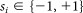are updated asynchronously as follows. At each time step t, a node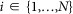is drawn uniformly. The field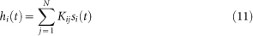is calculated. The state of node i is flipped with probability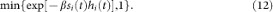Flipping the state of node i means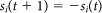. Otherwise the state of node i remains unchanged. All other nodes ji retain their state,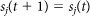.

The parameter β (inverse temperature) controls the order in the system. For large β (small temperature), spins tend to align and there is long-range order seen as large clusters of nodes sharing the same spin value. For small β (high temperature), long-range order is absent. The magnetization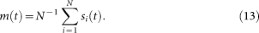is used to quantify the order of the system. Disordered systems have 〈|m|〉 ≈ 0, while a finite positive value is obtained in ordered systems.

### Rank order correlation

For a vector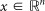, the rank of component i is given by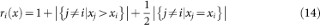The rank order correlation coefficient ρ(x, y) between two such vectors x and y is the Pearson correlation coefficient between the rank vectors r(x) and r(y). Thus ρ(x, y) takes values in [−1, 1] withif and only if x and y are in a strictly increasing (decreasing) relation.

### Degree and degree ratio

The degree di of node i is the number of nodes i is connected to. In directed networks, in- and out-degree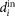and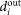are distinguished. For the matrix averaging over all adjacency matrices of networks with fixed node degrees, ci = di is a left eigenvector for the largest eigenvalue. Likewise, the degree ratios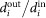form a left eigenvector of the Laplacian matrix averaging over all networks with given node degrees48,49.

## References

• Dorogovtsev, S. N. & Mendes, J. F. F. Evolution of networks: From biological nets to the Internet and WWW (Oxford University Press, Oxford, 2003).

• Albert, R. & Barabási, A.-L. Statistical mechanics of complex networks. Rev. Mod. Phys. 74, 47–97 (2002).

• Pastor-Satorras, R. & Vespignani, A. Epidemic spreading in scale-free networks. Physical Review Letters 86, 32003203 (2001).

• Barabási, A.-L. & Albert, R. Emergence of scaling in random networks. Science 286, 509–512 (1999).

• Jeong, H., Mason, S. P., Barabási, A.-L. & Oltvai, Z. N. Lethality and centrality in protein networks. Nature 411, 41–42 (2001).

• Wuchty, S. & Stadler, P. F. Centers of complex networks. Journal of Theoretical Biology 223, 45–53 (2003).

• Liu, Y.-Y., Slotine, J.-J. & Barabási, A.-L. Controllability of complex networks. Nature 43, 123–248 (2011).

• Freeman, L. A set of measures of centrality based upon betweenness. Sociometry 40, 35–41 (1977).

• Bonacich, P. Factoring and weighting approaches to clique identification. Journal of Mathematical Sociology 2, 113–120 (1972).

• Bollobás, B. Graph Theory and Combinatorics: Proceedings of the Cambridge Combinatorial Conference in Honor of P. Erds Vol. 35 (Academic, 1984).

• Seidman, S. B. Network structure and minimum degree. Social Networks 5, 269 (1983).

• Dorogovtsev, S. N., Goltsev, A. V. & Mendes, J. F. F. k-core organization of complex networks. Phys. Rev. Lett. 96, 040601 (2006).

• Kitsak, M. et al. Identifying influential spreaders in complex networks. Nature Physics 6, 888893 (2010).

• Masuda, N., Kawamura, Y. & Kori, H. Impact of hierarchical modular structure on ranking of individual nodes in directed networks. New Journal of Physics 11, 113002 (2009).

• Masuda, N. & Kori, H. Dynamics-based centrality for directed networks. Phys. Rev. E 82, 056107 (2010).

• Golub, B. & Jackson, M. O. Nave learning in social networks and the wisdom of crowds. American Economic Journal: Microeconomics 2, 112–49 (2010).

• Brin, S. & Page, L. The anatomy of a large-scale hypertextual Web search engine. Proceedings of the Seventh International World Wide Web Conference, Computer Networks and ISDN Systems 30, 107–117 (1998).

• Mises, R. V. & Pollaczek-Geiringer, H. Praktische verfahren der gleichungsauflösung. ZAMM - Journal of Applied Mathematics and Mechanics/Zeitschrift für Angewandte Mathematik und Mechanik 9, 58–77 (1929).

• Dorogovtsev, S. N., Goltsev, A. V. & Mendes, J. F. F. Critical phenomena in complex networks. Rev. Mod. Phys. 80, 1275–1335 (2008).

• Keeling, M. J. & Rohani, P. Modeling Infectious Diseases in Humans and Animals (Princeton University Press, 2007).

• Bansal, S., Grenfell, B. T. & Meyers, L. A. When individual behaviour matters: homogeneous and network models in epidemiology. Journal of The Royal Society Interface 4, 879–891 (2007).

• House, T. & Keeling, M. J. Insights from unifying modern approximations to infections on networks. Journal of The Royal Society Interface (2011).

• Rhodes, C. J. & Anderson, R. M. Power laws governing epidemics in isolated populations. Nature 381, 600–602 (1996).

• Pinto, O. A. & Muoz, M. A. Quasi-neutral theory of epidemic outbreaks. PLoS ONE 6, e21946 (2011).

• Harris, T. E. Contact interactions on a lattice. Ann. Probab. 2, 969–988 (1974).

• Christakis, N. A. & Fowler, J. H. Social network sensors for early detection of contagious outbreaks. PLoS ONE 5, e12948 (2010).

• Castellano, C. & Pastor-Satorras, R. Competing activation mechanisms in epidemics on networks. ArXiv e-prints (2011). 1105.5545.

• Borge-Holthoefer, J. & Moreno, Y. Absence of influential spreaders in rumor dynamics. ArXiv e-prints (2011). 1112.2239.

• Restrepo, J. G., Ott, E. & Hunt, B. R. Characterizing the dynamical importance of network nodes and links. Phys. Rev. Lett. 97, 094102 (2006).

• Ising, E. Beitrag zur theorie des ferromagnetismus. Zeitschrift für Physik A, Hadrons and Nuclei 31, 253–258 (1925).

• Brush, S. G. History of the lenz-ising model. Rev. Mod. Phys. 39, 883–893 (1967).

• Dorogovtsev, S. N., Goltsev, A. V. & Mendes, J. F. F. Ising model on networks with an arbitrary distribution of connections. Phys. Rev. E 66, 016104 (2002).

• Granovetter, M. Threshold models of collective behavior. American J Sociol 83, 14201443 (1978).

• González-Avella, J. C., Eguíluz, V. M., Marsili, M., Vega-Redondo, F. & San Miguel, M. Threshold learning dynamics in social networks. PLoS ONE 6, e20207 (2011).

• Schelling, T. Dynamic models of segregation. J. Math. Sociol. 1, 143–186 (1971).

• Vinkovic, D. & Kirman, A. A physical analogue of the schelling model. Proc. Natl. Acad. Sci. (USA) 103, 19261–19265 (2006).

• Blume, L. E. The statistical mechanics of strategic interaction. Games and Economic Behavior 5, 387–424 (1993).

• Klemm, K., Eguíluz, V. M., Toral, R. & San Miguel, M. Global culture: A noise induced transition in finite systems. Physical Review E 67, 045101(R) (2003).

• Hertz, J., Krogh, A. & Palmer, R. G. Introduction to the Theory of Neural Computation (Addison-Wesley, 1991).

• Agaev, R. & Chebotarev, P. On the spectra of nonsymmetric laplacian matrices. Linear Algebra and its Applications 399, 157–168 (2005). Special Issue devoted to papers presented at the International Meeting on Matrix Analysis and Applications, Ft. Lauderdale, FL, 14-16 December 2003.

• Holley, R. & Liggett, T. Ergodic theorems for weakly interacting infinite systems and the voter model. Annals of Probability 3, 643–663 (1975).

• Suchecki, K., Eguíluz, V. M. & San Miguel, M. Conservation laws for the voter model in complex networks. Europhysics Letters 69, 228–234 (2005).

• Mobilia, M. Does a single zealot affect an infinite group of voters? Phys. Rev. Lett. 91, 028701 (2003).

• Acebrón, J. A., Bonilla, L. L., Pérez Vicente, C. J., Ritort, F. & Spigler, R. The kuramoto model: A simple paradigm for synchronization phenomena. Reviews of Modern Physics 77, 137–185 (2005).

• Honey, C. J., Kötter, R., Breakspear, M. & Sporns, O. Network structure of cerebral cortex shapes functional connectivity on multiple time scales. Proceedings of the National Academy of Sciences of the USA 104, 10240–10245 (2007).

• Kauffman, S. A. The Origins of Order (Oxford University Press, New York, 1993).

• Ghanbarnejad, F. & Klemm, K. Impact of individual nodes in Boolean network dynamics. ArXiv e-prints (2011). 1111.5334.

• Serrano, M. A., Klemm, K., Vazquez, F., Eguíluz, V. M. & San Miguel, M. Conservation laws for voter-like models on random directed networks. Journal of Statistical Mechanics: Theory and Experiment 2009, P10024 (2009).

• Masuda, N. & Ohtsuki, H. Evolutionary dynamics and fixation probabilities on directed networks. New Journal of Physics 11, 033012 (2009).

• Zachary, W. W. An information flow model for conflict and fission in small groups. Journal of Anthropological Research 33, 452–473 (1977).

• Girvan, M. & Newman, M. E. J. Community structure in social and biological networks. Proceedings of the National Academy of Sciences of the USA 99, 7821–7826 (2002).

• Guimerà, R., Danon, L., Díaz-Guilera, A., Giralt, F. & Arenas, A. Self-similar community structure in a network of human interactions. Phys. Rev. E 68, 065103 (2003).

• Watts, D. J. & Strogatz, S. H. Collective dynamics of small-world networks. Nature 393, 440–442 (1998).

• White, J. G., Southgate, E., Thomson, J. N. & Brenner, S. The Structure of the Nervous System of the Nematode Caenorhabditis elegans. Philosophical Transactions of the Royal Society of London. B, Biological Sciences 314, 1–340 (1986).

## Acknowledgements

K.K. acknowledges financial support from VolkswagenStiftung and from European Commission NEST Pathfinder initiative on Complexity through project SYNLET (Contract 043312). M.A.S. acknowledges financial support by the Ramón y Cajal program of the Spanish Ministry of Science, MICINN Project No. BFU2010-21847-C02-02 and Generalitat de Catalunya grant No. 2009SGR1055. V.M.E. and M.S.M. acknowledge financial support from MICINN (Spain) and FEDER (EU) through projects FISICOS (FIS2007-60327) and MODASS (FIS2011-24785).

## Author information

Authors

### Contributions

K.K. contributed the computational results. K.K., M.A.S., V.M.E. and M.S.M. planned the reasearch and wrote and reviewed the manuscript.

## Ethics declarations

### Competing interests

The authors declare no competing financial interests.

## Electronic supplementary material

### Supplementary Information

supplementary info

## Rights and permissions

Reprints and Permissions

Klemm, K., Serrano, M., Eguíluz, V. et al. A measure of individual role in collective dynamics. Sci Rep 2, 292 (2012). https://doi.org/10.1038/srep00292

• Accepted:

• Published:

• DOI: https://doi.org/10.1038/srep00292

• ### Influence control method on directed weighted signed graphs with deterministic causality

• Alexander Tselykh
• Fernando A. F. Ferreira

Annals of Operations Research (2022)

• ### UACD: A Local Approach for Identifying the Most Influential Spreaders in Twitter in a Distributed Environment

• Md Saiful Islam

Social Network Analysis and Mining (2022)

• ### Best influential spreaders identification using network global structural properties

• Amrita Namtirtha
• Animesh Dutta
• Yogesh Simmhan

Scientific Reports (2021)

• ### Algorithm for determining the mutual impact of nodes in weighted directed graphs

• Dmytro Lande
• Oleh Dmytrenko
• Andrei Snarskii

Soft Computing (2021)

• ### Identification of effective spreaders in contact networks using dynamical influence

• Ruaridh A. Clark
• Malcolm Macdonald

Applied Network Science (2021)